• 目的：由左图实现图例部分字型修改，如右图 图标legend('')中部分字体设置：正常编码出图后，选中legend框右击选择字型，找到自己想要的字体，记住字型名字。 回到图形legend框选中你想要改字型的字，将其删除，在...
目的：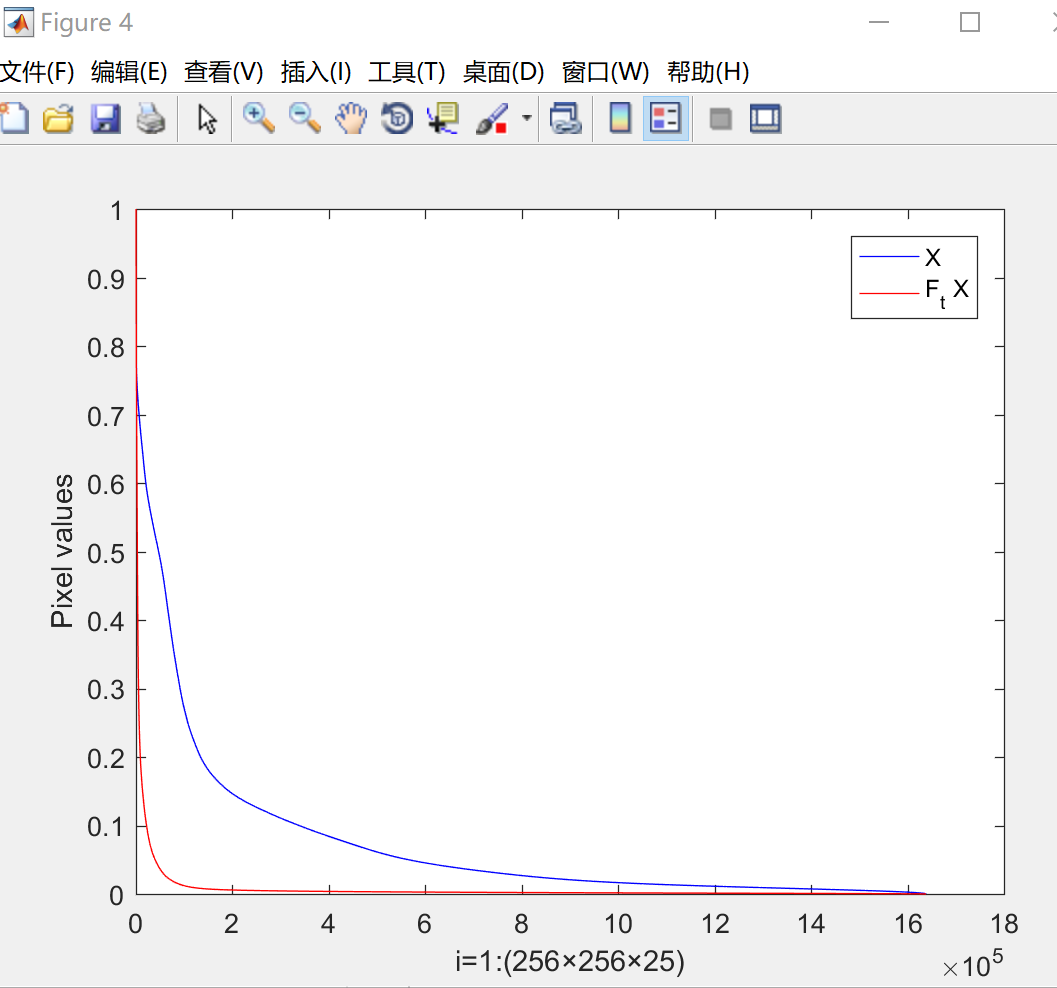由左图实现图例部分字型修改，如右图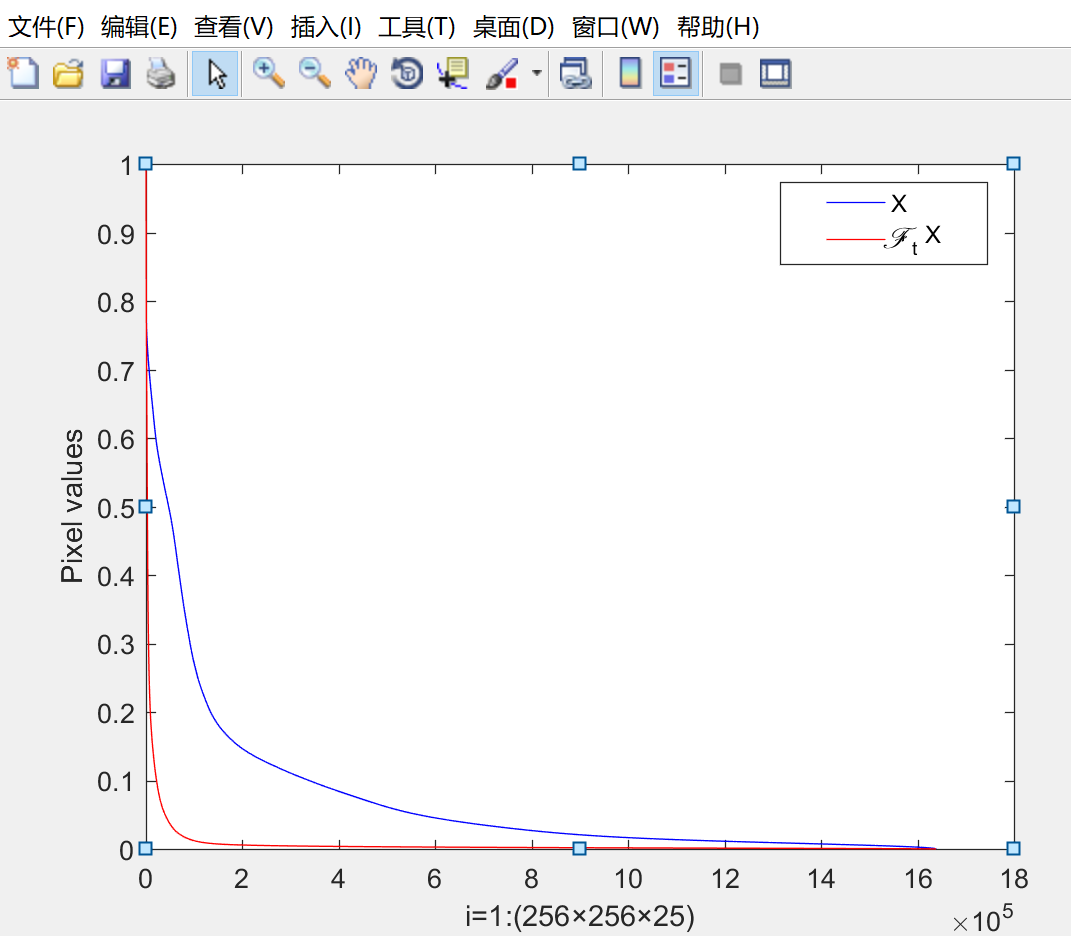图标legend('')中部分字体设置：正常编码出图后，选中legend框右击选择字型，找到自己想要的字体，记住字型名字。
回到图形legend框选中你想要改字型的字，将其删除，在图上方列表里选择‘插入’，‘文本框’构建一个文本输入你要改字型的字，然后选中文本框，右击选择字型（刚记住的字型名字），然后选择‘线型’无，将此文本框拖动覆盖到原legend位置处，完成。

具体操作如下：

示例代码：figure
plot( 1:length(sp1),sp0,'-b',1:length(sp1),sp1,'-r')
legend('X','F_t X')%%Lucida Calligraphy字型设置Script MT Bold字体
% Palace Script MT字体Kunstler Script字体
ylabel('Pixel values')
xlabel('i=1:(256×256×25)')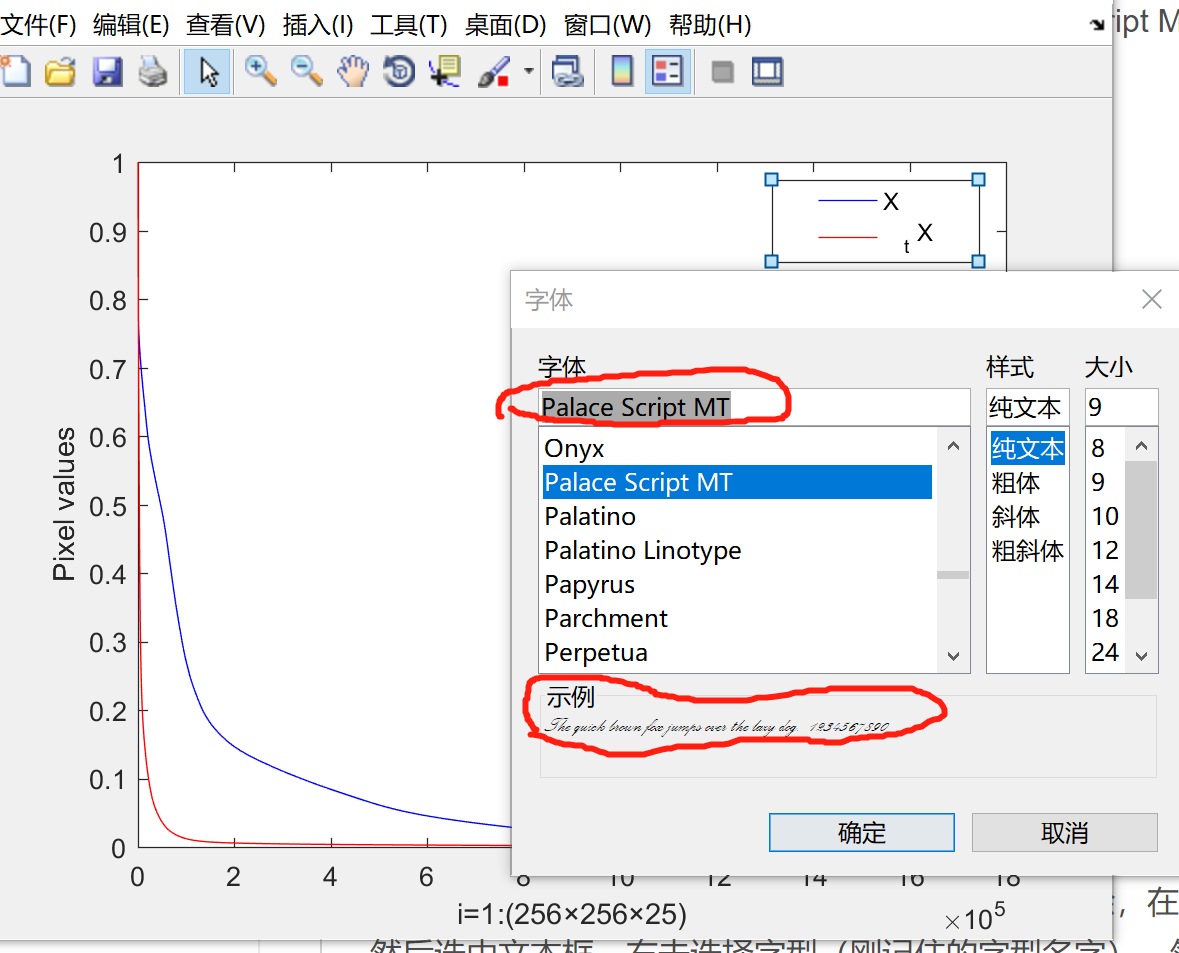想要改图例legend中字'F'为花体,现将F删除，并留出空格，选出你想要的字型，可以右击图例框，‘字型’里根据‘示例’的样子找到你想要的字型，记住名字。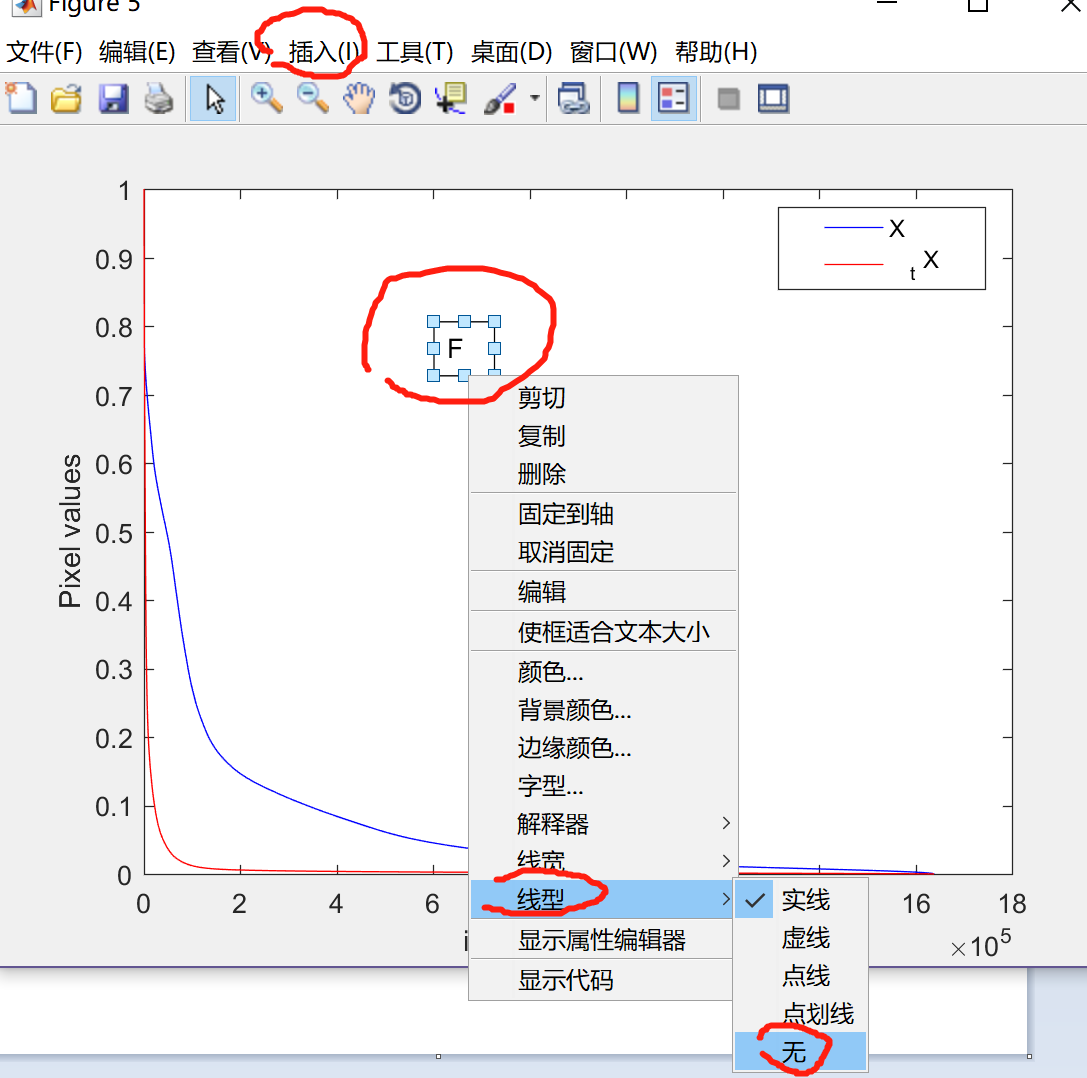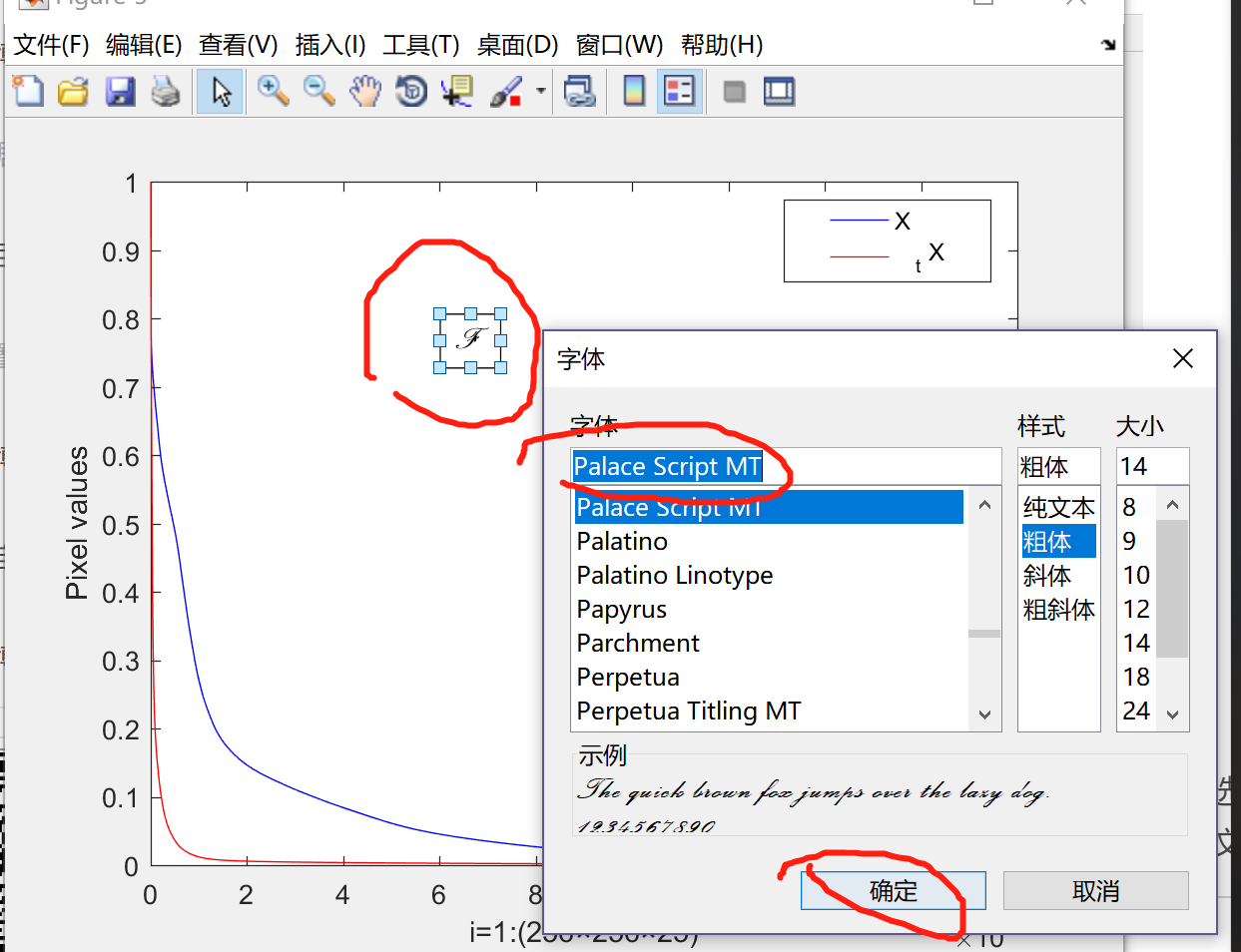然后关闭，在图上方选‘插入’ ‘文本框’ 输入'F',然后右击文本框 设置‘线型’为‘无’，再设置字型为刚才记住的字型名字，将文本框拖动到图例中合适的位置。保存为png图或者fig等。OK


展开全文• matlab 画图时，发现线太多，生成的图例，遮盖了曲线。于是想画成多个图例，然后可以自由拖动。  废话不多说，2）代码（设置多个图例部分在最后20行）； 1）效果。
     用matlab 画图时，发现线太多，生成的图例，遮盖了曲线。于是想画成多个图例，然后可以自由拖动。
废话不多说，2）代码（设置多个图例的部分在最后20行）； 1）效果。

%  ----- 1 效果: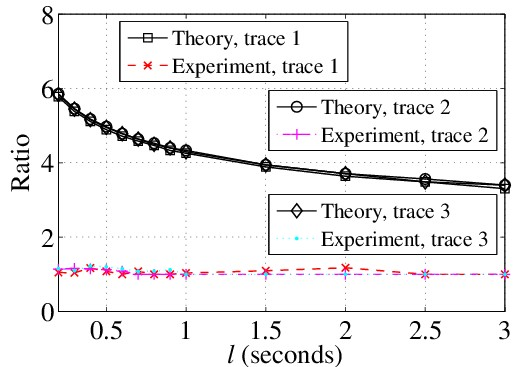%  ----- 2 关键部分代码：

% ===== Figure format :
figure
TextFontSize=20;
LegendFontSize = 16;
set(0,'DefaultAxesFontName','Times',...
'DefaultLineLineWidth',1,...
'DefaultLineMarkerSize',8);
set(gca,'FontName','Times New Roman','FontSize',TextFontSize);
set(gcf,'Units','inches','Position',[0 0 6.0 4.0]);
% ===== Figure format :~

% ============================== Plot the Trace1 :
% --- 1
h11 = plot(X_items1, UB_ratio_Ana_GA1, '-sk');
hold on
% --- 2
h12 = plot(X_items1, Ratio_GA_over_OPT1, '--xr');
hold on
% === Plot the Trace1 :~

% ============================== Plot the Trace2 :
% --- 1
h21 = plot(X_items2, UB_ratio_Ana_GA2, '-ok');
hold on
% --- 2
h22 = plot(X_items2, Ratio_GA_over_OPT2, '-.+m');
hold on
% === Plot the Trace2 :~

% ============================== Plot the Trace3 :
% --- 1
h31 = plot(X_items3, UB_ratio_Ana_GA3, '-dk');
hold on
% --- 2
h32 = plot(X_items3, Ratio_GA_over_OPT3, ':.c');
hold on
% === Plot the Trace3 :~

grid on
axis([0.2 3.0 0 8])

xlabel('{\it l} (seconds)')
ylabel('Ratio')

% ============= Legend 1 :
hg1 = legend('Theory, trace 1', 'Experiment, trace 1', 0);
set(hg1,'FontSize',LegendFontSize);
% === Legend 1 :~

ah2 = axes('position',get(gca,'position'),'visible','off');

% ============= Legend 2 :
hg2 = legend(ah2, [h21 h22], 'Theory, trace 2', 'Experiment, trace 2', 0);
set(hg2,'FontSize',LegendFontSize);
% === Legend 2 :~

ah3 = axes('position',get(gca,'position'), 'visible','off');

% ============= Legend 3 :
hg3 = legend(ah3, [h31 h32], 'Theory, trace 3', 'Experiment, trace 3', 0);
set(hg3,'FontSize',LegendFontSize);
% === Legend 3 :~

Davy_H
2014-9-13

展开全文• h1=plot(x1,y1); h2=plot(x2,y2);h3=plot(x3,y3); h4=plot(x4,y4);...四线，图例仅显示1，3 %%%%%%%%%%%%%%%%%%%%%%%%%%%%% %生成25*25矩阵 data = rand(25)+repmat(1:25,25,1); H ...
h1=plot(x1,y1);
h2=plot(x2,y2);h3=plot(x3,y3);
h4=plot(x4,y4);
legend([h1 h3],'x1-y1','x3-y3');
四线，图例仅显示1，3

%%%%%%%%%%%%%%%%%%%%%%%%%%%%%

%生成25*25矩阵

data = rand(25)+repmat(1:25,25,1);

H = plot(data);

%只对其中的部分曲线进行标注

h1=legend(H([1 6 11 16 21]),'1','6','11','16','21')

%使得标注横向显示

set(h1,'Orientation','horizon')

%去除标记框的外围长方形

set(h1,'Box','off');

%%%%%%%%%%%%%%%%%%%%%%%%%%

方法1：复杂到吐血

例如你有25条曲线，想显示其中1，6，11，16，21的legend，则

for i = [2:5 7:10 12:15 17:20 22:25]
set(get(get(H(i),'Annotation'),'LegendInformation'),'IconDisplayStyle','off');
end
legend('1','6','11','16','21');

方法2：简单到郁闷

H = plot(data);
legend(H([1 6 11 16 21],'1,'6','11’,'16','21');

如果一个图中我们画了n条曲线，但是我们只想加图例说明（legend）的只有m条 （m

1. 最简单，最超级无敌的方法：把想要标注的图形命令给个变量名，然后再legend命令中指定。

x = -3.14:0.1:3.14;
y1 = sin(x);
y2 = cos(x);
y3 = .1*exp(x);
y4 = y1.*y3;
hold on
h1 = plot(x, y1, 'r');
h2 = plot(x, y2, 'g');
h3 = plot(x, y3, 'k');
h4 = plot(x, y4, 'm');
hold off
xlim auto
legend([h1,h3],'sin', 'exp');

2.通过控制Annotation属性来实现，详细控制方法参见http://www.mathworks.cn/help/techdoc/creating_plots/braliom.html;jsessionid=HPs0TNGQxP2TXMcZgQv4zkMvmSsZYbhG6Lwjd3JT271PLqXnHxhY!-1484299157。但是需要注意的是在稍微低版本的MATLAB中，并不提供Annotation的控制（至少我的R14SP1不支持）。

x = -3.14:0.1:3.14;
y1 = sin(x);
y2 = cos(x);
y3 = .1*exp(x);
y4 = y1.*y3;
hold on
h1 = plot(x, y1, 'r');
h2 = plot(x, y2, 'g');
h3 = plot(x, y3, 'k');
h4 = plot(x, y4, 'm');
hold off
xlim auto
set(get(get(h2, 'Annotation'), 'LegendInformation'), 'IconDisplayStyle', 'off');
set(get(get(h4, 'Annotation'), 'LegendInformation'), 'IconDisplayStyle', 'off');
legend('sin', 'exp');

3.多个legend以及标注部分图列构成多列图列

t=0:pi/100:2*pi;
y1=sin(t);
y2=cos(t);
y3=y1.*y2;
y4=0.5*(y1+y2);
hold on
h1=plot(t,y1,'-r')
%h11 = plot(t(1:10:end),y1(1:10:end),'o','MarkerFaceColor','r','MarkerEdgeColor','r');
h11 = plot(t(1:20:end),y1(1:20:end),'ro');
h2=plot(t,y2,'b-');
%h22 = plot(t(1:10:end),y2(1:10:end),'^','MarkerFaceColor','b','MarkerEdgeColor','b');
h22 = plot(t(1:20:end),y2(1:20:end),'b^')
h3=plot(t,y3,'c');
h4=plot(t,y4,'g');
hold off
[legh,objh,outh,outm]=legend([h1,h2],'y1','y2',1);
legend boxoff

% matlab 6.5.1
%set(objh(3),'marker','*');
%set(objh(5),'marker','.');

% matlab7
set(objh(4),'marker','o');
set(objh(6),'marker','^');

legh2=copyobj(legh,gcf);
[legh2,objh2]=legend([h3,h4],'y3','y4',2);
legend boxoff

这样画好后，只有第二个legend可拖动，而第一个legend不可拖动，原因不明。

4.Matlab提供的legend函数，给出的legend经常覆盖了某些曲线，这样就需要把legend分成几个，相对独立，这样可以使用鼠标随意移动，确保不遮挡曲线。

a=linspace(0,2*pi,100);
y1=100*sin(a);
y2=50*cos(a);
y3=tan(a);
y4=log(a);
y=[y1;y2;y3;y4];
figure
p=plot(a,y)
legend(p(1:2),'sin','cos');
ah=axes('position',get(gca,'position'),...
'visible','off');
legend(ah,p(3:4),'tan','log','location','west');

5.用plot函数对两个长度为30的向量分别绘制曲线的时候，在两条曲线上各画了一个marker（因为如果把所有的marker都放上去的话，感觉很拥挤，不是很好看），在对最终的画图效果做legend标注的时候，我希望将曲线及其上的marker一起标注

clc;
clear;
close all;

figure_handle = figure;
set(figure_handle, 'Color', 'w');
data=zeros(1, 100);
data(1) = 1;
data(2) = 1;
data(3) = 1;
t = [1 : 30] / 100 * 2 * pi;
cur1=real(fft(data) / 3);
cur1p = cur1(1 : 30);
h_1(1) = plot(t, cur1p, 'LineStyle', '-', 'LineWidth', 2, 'Color', 'r');
hold on
h_1(2) = plot(t(15), cur1p(15), 'LineStyle', '-', 'LineWidth', 2,  'Color', 'r', 'Marker', 's', 'MarkerSize', 10, 'MarkerEdgeColor', 'r', 'MarkerFaceColor', 'r');
set(h_1, 'Parent', hSGroup);
set(get(get(hSGroup, 'Annotation'), 'LegendInformation'), 'IconDisplayStyle', 'on');
cur2 = real(fft(data)) .* real(fft(data))/9;
cur2p = cur2(1 : 30);
h_2(1) = plot(t, cur2p, 'LineStyle', '-', 'LineWidth', 2, 'Color', 'g');
h_2(2) = plot(t(12), cur2p(12), 'LineStyle', '-', 'LineWidth', 2, 'Color', 'g', 'Marker', 'o', 'MarkerSize',10, 'MarkerEdgeColor', 'g', 'MarkerFaceColor', 'g');
set(h_2, 'Parent', hCGroup);
set(get(get(hCGroup, 'Annotation'), 'LegendInformation'), 'IconDisplayStyle', 'on');
legend('1 level', '2 levels');


展开全文• cell_string{2}='\\fontsize{16} \\font...在matlab中为图形加标注以及文字说明的方法技巧想要修改图例中的文字说明,就直接在说明部分双击后,会出现文字编辑框,这个时候直接输入图例文字就可以. 注意事项 论文作图...cell_string{2}='\\fontsize{16} \\fontname{隶书}镇定时间';   绘制图形如下图:。如何在Matlab画图中加入字符等图形标示。在matlab中为图形加标注以及文字说明的方法技巧。在matlab中为图形加标注以及文字说明的方法技巧想要修改图例中的文字说明,就直接在说明部分双击后,会出现文字编辑框,这个时候直接输入图例文字就可以.   注意事项   论文作图要严谨明显,学生朋友们要多练习尝试   图例的位置也就需要做适当的调整,此时可以通过右键点击图例选择location,选择不同的。Matlab作图怎么修改编辑图例4、图形保持   一般情况下,每执行一次命令就刷新当前窗口,图形窗口原有图形将不复存在.若希望在已存在的图形上再继续添加新的图形,可以使用图形保持命令hold.   hold on保持   hold off取消保持   hold 在两者之间切换。MATLAB学习笔记 五 MATLAB绘图7、按钮画图函数添加代码:   函数代码的意义涉及到COM,正在写VC调用COM组件所涉及到的有关COM方面的知识.   8、设置预编译头文件:工程->设置(快捷键Alt+F7),选择C/C++项precomplied Headers,设置如图11   6。图9 添加头文件-浅析VC与Matlab联合编程 三展开全文• 1. 插入图例的时候，如果仅保留部分，可以使用 legend([],'variable1','variable2')，'variable1'为第一个数据的名字，不要的图例使用[]代替 2. 修改X/Y轴的起始点和代号 红框的Auto要打掉勾，至于显示什么在...
• 说明 部分代码写法不是规范写法，为了表达意思可能会有伪代码，能看懂就行 基本命令 & 图例 plot图 scatter图 其他图 曲面 &... 图例 ...Matlab官方文档关于图例和一些辅助命令的使用：h...科学计算
• 1部分分式展开法求F(s)的反变换利用MATLAB分析LTI系统的特性例6-2画出H(s)零极点并判断稳定性利用MATLAB 进行 Laplace 正、反变换例6-3Laplace正反变换离散系统零极点图例6-4函数零极点图离散系统的频率特性例6-5取...
• 1. 插入图例的时候，如果仅保留部分，可以使用legend([],'variable1','variable2')，'variable1'为第一个数据的名字，不要的图例使用[]代替2. 修改X/Y轴的起始点和代号红框的Auto要打掉勾，至于显示什么在“Ticks”...
• 1.legend('string1','string2',…)用指定的文字string在当前坐标轴中对所给数据的每一部分显示一个图例。 2.legend(h,'string1','string2',…)用指定的文字string在一个包含于句柄向量h中的图形显示图例。用给定的...
• 部分内容摘自：...legend 是matlab中用于设置图例的线条和patches的函数，例如下图右上角上的线条和字幕的设置等等。 函数使用方法： legend('string1','string2',...)  legend(h,'string1','string2',...
• 冈萨雷斯数字图像处理(matlab版),这是图像处理基础理论论述同以MATLAB为主要工具的软件实践方法相对照的第一本书。本书集成了冈萨雷斯和伍兹所著的《数字图像处理》一...配套经典的冈萨雷斯数字图像处理中的源码和图例
• 以下内容为个人笔记，部分图片来源于郭老师课件或课程截图。 笔记汇总：MATLAB基础教程 课程视频：MATLAB基础教程-台大郭彦甫（14课全-高清-含课件） 文章目录基础绘图plot() 基本使用plot() 描点、连线legend() ...数据可视化
• 目录 legend 在作图命令中（plot）给出图例标签； legend(label1,...,labelN) ...给部分函数添加图例； legend(___,'Location',lcn) 指定图例的位置（方向）以及显示的列数； lgd = legend(___)...
• 问题：如何在Matlab中，将图例绘制成多行多列的？ 查找资料过程中发现， 1）有的方法要求多个plot中横坐标具有相同的长度 比如：https://blog.csdn.net/xgf415/article/details/56480011 2）高版本中，部分函数...legend
• 提供的下载资源“MATLAB中pettitt突变检测与绘图”代码中，图例存在bug。请按照以下加粗部分代码修改。 p1 = plot(data(2:m,1),U,'k-','linewidth',1.5);%画出整个曲线的变化趋势p2 = plot([data(loc+1,1),data...
• 绘制了多条曲线，但只想图例指出其中的一部分。 如下图，只想指出红色和蓝色曲线： 尝试方法（失败） 分别在红蓝两部分绘画结束后，加两个legend（） 在第一部分绘画结束后加legend，第二部分加如下语句： plot...
• 最近遇到一个问题，用matlab绘制一堆线和点之后，想添加一下图例，但是遇到两个新问题 第一个是，需要绘制的图例比较多，用一个句子表达起来很长很长，除了不美观之外，致命的问题是这种方法太笨； 第二个问题...legend 学习
• 今天浏览了Matlab官网，看了一下官网提供的Matlab的绘图工具箱，因为很多之前没有注意到，部分虽然自己可以实现，但是功能不够完善，因此，今天做一下工具箱的总结，以用来记录自己的学习过程，详情请移步MathWorks...
• 由于是小组合作,这里就只发布我做的第一部分,裁剪线的删除和增加部分,以及GUI部分不做叙述. 如上，在这张图中，明显重要的内容就是飞鸟，而背景则不重要。传统的缩放技术，一是等比例缩放，如果纵向和横线缩放的...数字图像
• 本文主要是两类数据用散点图作比较时候的绘制方法，自己做时候在加图例这一步骤上尝试了很多次，因而写本文做记录、分享。 绘制方法写在见面plotscattergscatter 写在见面 emmm，简要的写一个可以应用的背景哈...
• 坐标轴的调整和图像标注7.1坐标轴调整命令7.2图形标注7.2.1坐标轴和图像标题标注7.2.2图例的标注7.2.3控制分格线结尾的话 5.特殊图像绘制 5.1 面积图area 表现各个不同部分对整体所作的贡献 命令格式： area(X,Y)：...可视化 数据可视化
• 一般的原则就是开门见栓，平面图中消防箱图例空白三角尖为门锁位置，空白三角的短边就是门轴，黑色三角短边部分就是栓头；面对消防箱门，从左向右开即为右开，反之为左开。下面拿实例说明一下。如图一：这是比较正规...
• 您可以单独运行每个部分，也可以一次运行所有代码。 第一次运行时，我建议“运行整个代码”。 然后，您可以更改图形，变量或您想要的任何内容 对于敏感性测试，请使用文件sensianalysis.m。 这使用了sensitivity....# matlab图例部分matlab 订阅